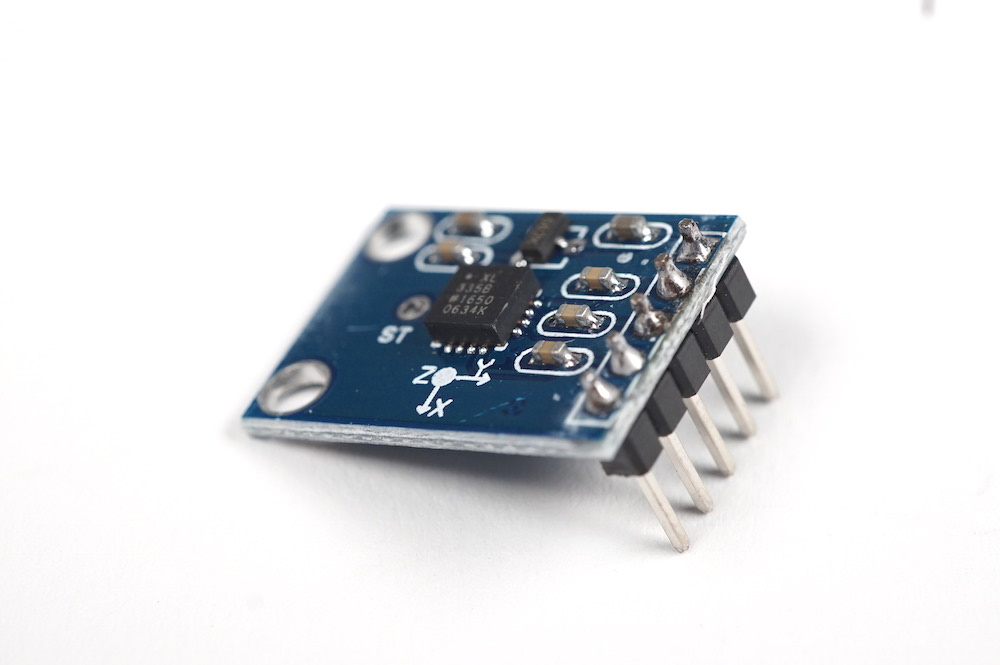3-Axis Accelerometer
Category:
Subcategory:
Signal Type:
Continuous (many possible values)
IDeATe Component Number:
0368
Senses acceleration in X, Y, and Z axes; used to detect motion and orientation.What it is

The three-axis accelerometer measures acceleration in the X, Y, and Z axes.

When to use it

When you need to measure acceleration, orientation, or physical movement of the sensor.

How it works

This sensor works by housing a Micro Electro-Mechanical System (MEMS) inside of the microchip. Springs suspend floating fingers in between fixed fingers. The floating fingers can move back and forth on one axis. Deflection in one direction or the other causes a change in capacitance between the floating and fixed fingers. This change in capacitance represents acceleration on one axis. There is a system of fingers for all the three axes.Image from authors Mukhiya and Pant via Wikimedia Commons
How to use it

The sensor is very easy to wire; 5 volts to `VCC`, ground to `GND`, and feed each of the outputs to one of the analog input lines on the Arduino. All three axes are measured simultaneously. If you only want to use it to measure acceleration in one or two axes, you may simply only wire the ones you want to read.

Getting startedFor this example, the accelerometer is connected to pins A0, A1, and A2
``````/*
* This program reads an accelerometer connected to ACCEL_X_PIN,
* ACCEL_Y_PIN, and ACCEL_Z_PIN and sends the data back to the
* computer via serial.
*
* Created 2021-02-19 by Perry Naseck
*/

const int ACCEL_X_PIN = A0; // Analog input pin for X movement
const int ACCEL_Y_PIN = A1; // Analog input pin for Y movement
const int ACCEL_Z_PIN = A2; // Analog input pin for Z movement

// Variables to keep track of the current positions
int accelXPos = 0;
int accelYPos = 0;
int accelZPos = 0;

void setup() {
// Setup serial port to send the accelerometer data back to the computer
Serial.begin(9600);

// Setup the accelerometer inputs
pinMode(ACCEL_X_PIN, INPUT);
pinMode(ACCEL_Y_PIN, INPUT);
pinMode(ACCEL_Z_PIN, INPUT);
}

void loop() {
// Get the current states

// Send the data over serial
Serial.print("X: ");
Serial.print(accelXPos);
Serial.print(" Y: ");
Serial.print(accelYPos);
Serial.print(" Z: ");
Serial.println(accelZPos);

// Delay to not send messages too fast
delay(100);
}
``````
Resources
Related Components